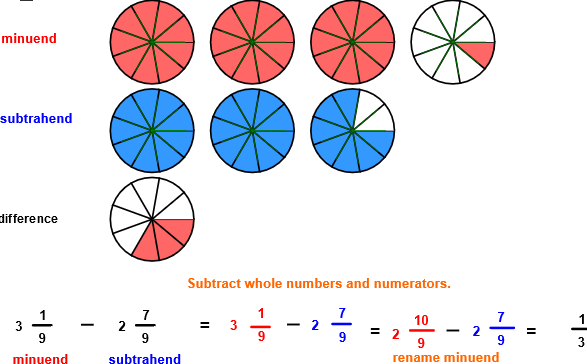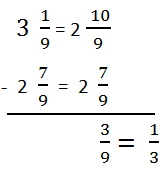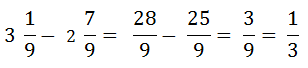# Subtract like fractions

## Subtract Like Fractions with Circle Models

SUBTRACT LIKE FRACTIONS
CORRECT:
ATTEMPTS:
SCORE:
PERCENT
WHOLE
NUMERATOR
DENOMINATOR

# SUBTRACT LIKE FRACTIONS INSTRUCTIONS

Follow the directions in the dialog box after pressing the <START> button. The <EXPLAIN> button may be pressed after you enter the subtrahend to see how to do the example.

The parts of a subtraction example are the minuend, the subtrahend, and the difference.

When the program starts, you will be asked to identify the minuend in the first row and then the subtrahend in the second row. You will then be asked to find the difference

The following image was made by Subtract Fractions With Circle Models Designer:Both minuend and subtrahend in this program have the same denominator. Fractions with the same denominators are like fractions.

The picture shows that the minuend has 3 19 units and the subtrahend has 2 79 units. The difference is what is left after removing the blue circles in the second row from the circles in the first row. In many examples, you can arrive at the difference by looking at the picture. For example, after removing the two whole circles, you are left with 10/9 red circles. Removing the 79 circle from the 109 circle will leave 39 or 13 circle.

When the numerator of the subtrahend is larger than the numerator of the minuend you may rename the minuend to subtract. Some texts call the procedure "borrowing". To borrow, simply decrease the whole number 3 by 1. Then add 99 to 19 to get 109, giving the fraction 2 109.

Notice in the above image that 3 1/9 is renamed as 2 109 so that the numerators can be subtracted.

You may prefer to work vertically:Another method would be to write the minuend and subtrahend in fraction form and then subtract the numerators. The example would look like this:For more instruction on subtracting fractions go to How To Subtract Fractions.

After you enter the difference you may press the <REPORT> button. The report will ask for your name but you may submit a code for your name. This report will give the same results as on the dialog box. The report may be printed or e-mailed.